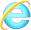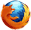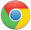# JavaScript random() 方法

2018-08-04 20:38 更新

## JavaScript random() 方法JavaScript Math 对象

## 实例

Math.random();

document.write(Math.random());

## 定义和用法

random() 方法可返回介于 0（包含） ~ 1（不包含） 之间的一个随机数。

## 浏览器支持Math.random()

## 返回值

Number 0.0 ~ 1.0（不包含） 之间的一个伪随机数。

## 技术细节

 JavaScript 版本： 1

## 实例

Math.floor((Math.random()*10)+1);

document.write(Math.floor((Math.random()*10)+1));

## 实例

Math.floor((Math.random()*100)+1);

document.write(Math.floor((Math.random()*100)+1));JavaScript Math 对象

App下载